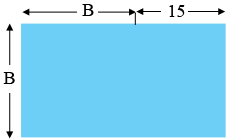SEARCH HOMEMath Central Quandaries & QueriesQuestion from Hazym, a student: How do I find the length(which is 15m longer than the breadth) and the breadth of a rectangle just by its perimeter which is 70 m?Hi Hazym,

I really find that a sketch helps.In my diagram $B$ is the breadth of the rectangle in metres. What is the perimeter of the rectangle in terms of $B?$ This perimeter is 70 metres. Solve for $B.$

PennyMath Central is supported by the University of Regina and the Imperial Oil Foundation.